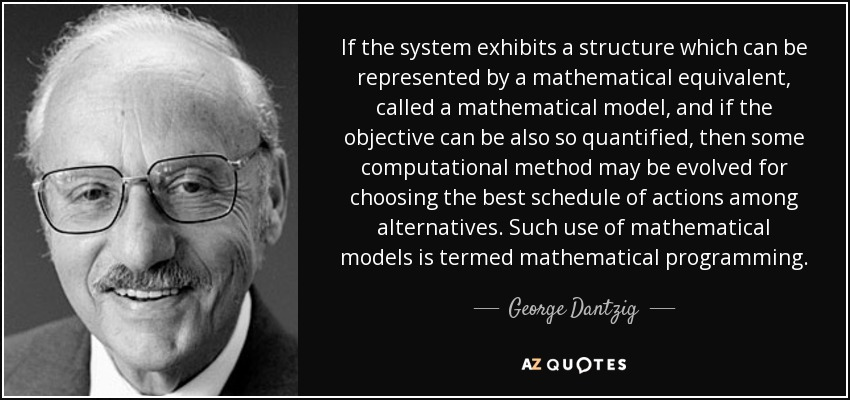Authors:
• If the system exhibits a structure which can be represented by a mathematical equivalent, called a mathematical model, and if the objective can be also so quantified, then some computational method may be evolved for choosing the best schedule of actions among alternatives. Such use of mathematical models is termed mathematical programming.

### TopicsEmbed: